• 常用的Excel函数公式大全
千次阅读
2021-06-30 00:39:53

Excel是工作中最常用的电脑办公工具之一。下面是学习啦小编为您精心整理的最常用的Excel函数公式大全。

篇一

零、随机数

1、随机数函数：

=RAND()

首先介绍一下如何用RAND()函数来生成随机数(同时返回多个值时是不重复的)。RAND()函数返回的随机数字的范围是大于0小于1。因此，也可以用它做基础来生成给定范围内的随机数字。

生成制定范围的随机数方法是这样的，假设给定数字范围最小是A，最大是B，公式是：=A+RAND()*(B-A)。

举例来说，要生成大于60小于100的随机数字，因为(100-60)*RAND()返回结果是0到40之间，加上范围的下限60就返回了60到100之间的数字，即=60+(100-60)*RAND()。

2、随机整数

=RANDBETWEEN(整数，整数)

如：=RANDBETWEEN(2,50)，即随机生成2~50之间的任意一个整数。

上面RAND()函数返回的0到1之间的随机小数，如果要生成随机整数的话就需要用RANDBETWEEN()函数了，如下图该函数生成大于等于1小于等于100的随机整数。

这个函数的语法是这样的：=RANDBETWEEN(范围下限整数，范围上限整数)，结果返回包含上下限在内的整数。注意：上限和下限也可以不是整数，并且可以是负数。

篇二

求和公式

1、隔列求和

公式：H3

=SUMIF($A$2:$G$2,H$2,A3:G3) =SUMPRODUCT((MOD(COLUMN(B3:G3),2)=0)*B3:G3) 说明：如果标题行没有规则用第2个公式 2、单条件求和 公式：F2 =SUMIF(A:A,E2,C:C) 说明：SUMIF函数的基本用法 3、单条件模糊求和 公式：详见下图 说明：如果需要进行模糊求和，就需要掌握通配符的使用，其中星号是表示任意多个字符，如"*A*"就表示a前和后有任意多个字符，即包含A。 4、多条件模糊求和 公式：C11 =SUMIFS(C2:C7,A2:A7,A11&"*",B2:B7,B11) 说明：在sumifs中可以使用通配符* 5、多表相同位置求和 公式：b2 =SUM(Sheet1:Sheet19!B2) 说明：在表中间删除或添加表后，公式结果会自动更新。 6、按日期和产品求和 公式：F2 =SUMPRODUCT((MONTH($A$2:$A$25)=F$1)*($B$2:$B$25=$E2)*$C$2:$C$25) 说明：SUMPRODUCT可以完成多条件求和 篇三 字符串处理公式 1、多单元格字符串合并 公式：c2 =PHONETIC(A2:A7) 说明：Phonetic函数只能对字符型内容合并，数字不可以。 2、截取除后3位之外的部分 公式： =LEFT(D1,LEN(D1)-3) 说明：LEN计算出总长度,LEFT从左边截总长度-3个 3、截取-前的部分 公式:B2 =Left(A1,FIND("-",A1)-1) 说明：用FIND函数查找位置，用LEFT截取。 4、截取字符串中任一段的公式 公式:B1 =TRIM(MID(SUBSTITUTE($A1," ",REPT(" ",20)),20,20))

说明:公式是利用强插N个空字符的方式进行截取

参加试验检测考试加微信:13527870418购买开通微试验题库

5、字符串查找

公式：B2

=IF(COUNT(FIND("河南",A2))=0,"否","是")

说明: FIND查找成功，返回字符的位置，否则返回错误值，而COUNT可以统计出数字的个数，这里可以用来判断查找是否成功。

6、字符串查找一对多

公式：B2

=IF(COUNT(FIND({"辽宁","黑龙江","吉林"},A2))=0,"其他","东北")

说明：设置FIND第一个参数为常量数组，用COUNT函数统计FIND查找结果

更多相关内容
• 今天和大家分享一组常用函数公式的使用方法，用心掌握这些函数，工作效率会大大提升。欢迎转发给更多有需要的人。一、相关概念(一)函数语法由函数名+括号+参数组成例： 求和函数：SUM(A1,B2,…) 。参数与参数之 间用...

今天和大家分享一组常用函数公式的使用方法，用心掌握这些函数，工作效率会大大提升。欢迎转发给更多有需要的人。

一、相关概念

(一)函数语法

由函数名+括号+参数组成

例： 求和函数：SUM(A1,B2,…) 。参数与参数之 间用逗号“,”隔开

(二)运算符

1. 公式运算符：加(+)、减(-)、乘(*)、除(/)、百分号(%)、乘幂(＾)

2. 比较运算符：大与(>)、小于(=) 、不等于(<>)

3. 引用运算符：区域运算符(：)、联合运算符(,)

(三)单元格的相对引用与绝对引用

例： A1

$A1 锁定第A列 A$1 锁定第1行

$A$1 锁定第A列与第1行

二、常用函数

(一)数学函数

1. 求和 =SUM(数值1,数值2,……)

2. 条件求和 =SUMIF(查找的范围,条件(即对象),要求和的范围)

例：(1)=SUMIF(A1:A4,”>=200”,B1:B4)

函数意思：对第A1栏至A4栏中，大于等于200的数值对应的第B1列至B4列中数值求和

(2)=SUMIF(A1:A4,”<300”,C1:C4)

函数意思：对第A1栏至A4栏中，小于300的数值对应的第C1栏至C4栏中数值求和

3. 求个数 =COUNT(数值1,数值2,……)

例：(1) =COUNT(A1:A4) 函数意思：第A1栏至A4栏求个数

(2) =COUNT(A1:C4) 函数意思：第A1栏至C4栏求个数

4. 条件求个数 =COUNTIF(范围,条件)

例：(1) =COUNTIF(A1:A4，”<>200”)

函数意思：第A1栏至A4栏中不等于200的栏求个数

(2) =COUNTIF(A1:C4,”>=1000”)

函数意思：第A1栏至C4栏中大于等1000的栏求个数

5. 求算术平均数 =AVERAGE(数值1,数值2,……)

例：(1) =AVERAGE(A1,B2)

(2) =AVERAGE(A1:A4)

6. 四舍五入函数 =ROUND(数值,保留的小数位数)

7. 排位函数 =RANK(数值,范围,序别) 1-升序 0-降序

例：(1) =RANK(A1,A1:A4,1)

函数意思：第A1栏在A1栏至A4栏中按升序排序，返回排名值。

(2) =RANK(A1,A1:A4,0)

函数意思：第A1栏在A1栏至A4栏中按降序排序，返回排名值。

8. 乘积函数 =PRODUCT(数值1,数值2,……)

9. 取绝对值 =ABS(数字)

10. 取整 =INT(数字)

(二)逻辑函数

条件函数：=IF(条件,执行条件,执行条件假)—可执行七层嵌套

例： A1=5 A2=3 A3=6 A4=7

=IF(A1>A2,1,0)=1(真)

=IF(A1

=IF(A1>A2,IF(A3>A4,8,9),1)=9

(三)文本函数

1. 截取函数： =LEFT(文本,截取长度)—从左边截取

=RIGHT(文本,截取长度)—从右边截取

=MID(文本,开始位,截取长度)—从中间截取

2. 计算字符长度：LEN(文本)—文本的长度

3. 合并字符函数：CONCATENATE(文本1,文本2,…)

4. 在字符串中查找特定字符：FIND(文本,范围,数值)—数值表示查找第几个

5. 比较两个字符是否完全相符：EXACT(文本1,文本2)

=EXACT(“a”,“a”)=TRUE

=EXACT(“a”,“ab”)=FALSE

=EXACT(“a”,“A”)=FALSE

6. 将数值转化为文本：TEXT(数值,参数) )—参数一般为0

7. 将数值型字符转换为数值：VALUE(数值文本)

(四)判断公式

1. 把公式产生的错误显示为空

公式：C2=IFERROR(A2/B2,””)

说明：如果是错误值则显示为空，否则正常显示。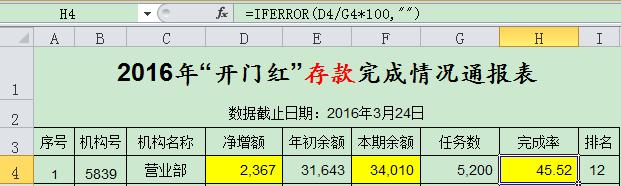2. IF多条件判断返回值

公式：C2=IF(AND(A2<500,B2=”未到期”),”补款”,””)

说明：两个条件同时成立用AND，任一个成立用OR函数。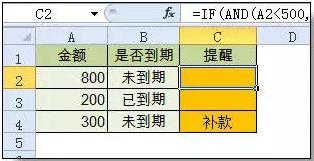(五)统计公式

1. 统计两个表格重复的内容

公式：B2=COUNTIF(Sheet15!A:A,A2)

说明：如果返回值大于0说明在另一个表中存在，0则不存在。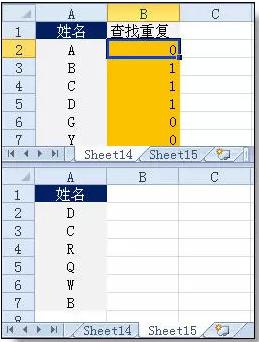2. 统计不重复的总人数

公式：C2=SUMPRODUCT(1/COUNTIF(A2:A8,A2:A8))

说明：用COUNTIF统计出每人的出现次数，用1除的方式把出现次数变成分母，然后相加。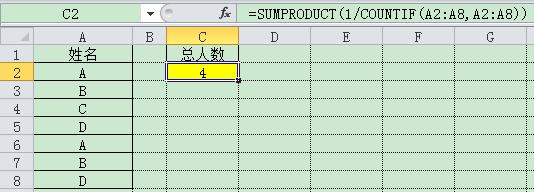(六)求和公式

1. 隔列求和

公式：H3=SUMIF($A$2: $G$2,H$2,A3:G3) 或者： =SUMPRODUCT((MOD(COLUMN(B3:G3),2)=0)*B3:G3) 说明：如果标题行没有规则用第2个公式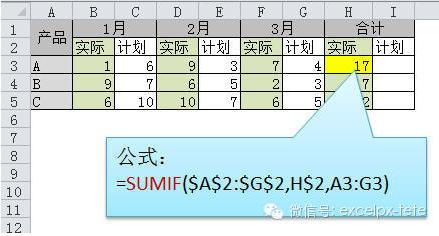2. 单条件求和 3. 单条件模糊求和 4. 多条件模糊求和 5. 多表相同位置求和 公式：B2=SUM(Sheet1:Sheet19!B2) 说明：在表中间删除或添加表后，公式结果会自动更新。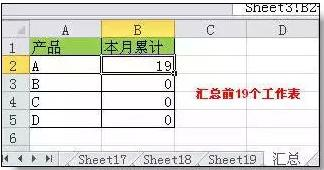6. 按日期和产品求和 (七)查找与引用公式 1. 单条件查找公式 公式1： C11=VLOOKUP(B11,B3:F7,4,FALSE) 说明：查找是VLOOKUP最擅长的，基本用法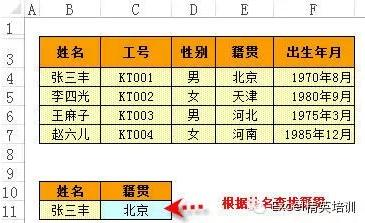2. 双向查找公式 公式=INDEX(C3:H7,MATCH(B10,B3:B7,0),MATCH(C10,C2:H2,0)) 说明：利用MATCH函数查找位置，用INDEX函数取值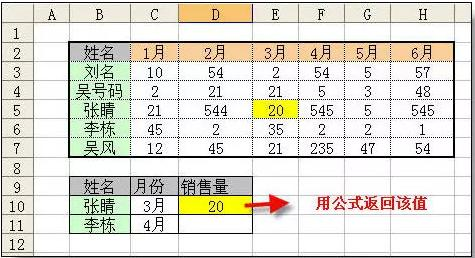3. 查找最后一条符合条件的记录。 公式：详见下图 说明：0/(条件)可以把不符合条件的变成错误值，而lookup可以忽略错误值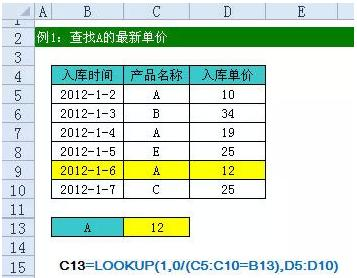4. 多条件查找 公式：详见下图 说明：公式原理同上一个公式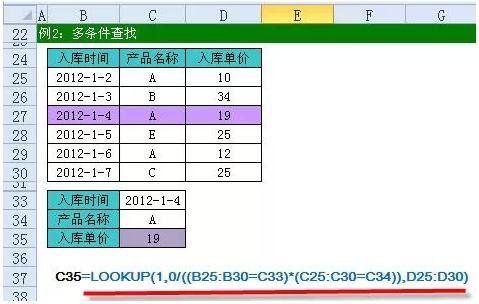5. 指定区域最后一个非空值查找 6. 按数字区域间取对应的值 展开全文• EXCEL常用函数公式大全与举例举列常用函数.doc文档 • EXCEL常用函数公式大全与举例-举列常用函数.doc文档 • excel常用函数公式大全：各种日期格式的转换公式.doc文档 • 原标题：十五个常用函数公式，值得收藏小伙伴好啊，今天和大家一起来学习几个常用函数的使用方法。1、判断销售额是否达标IF函数是最常用的判断类函数之一，能完成非此即彼的判断。如下图，考核得分的标准为9分，要... 原标题：十五个常用函数公式，值得收藏 小伙伴好啊，今天和大家一起来学习几个常用函数的使用方法。 1、判断销售额是否达标 IF函数是最常用的判断类函数之一，能完成非此即彼的判断。 如下图，考核得分的标准为9分，要判断B列的考核成绩是否合格。 =IF(B4>=9,"合格","不合格")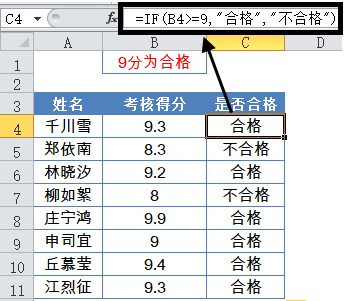IF，相当于普通话的“如果”，常规用法是： IF(判断的条件,符合条件时的结果,不符合条件时的结果) 2、按班级统计总成绩 如下图所示，使用SUMIF函数计算一班的总成绩： =SUMIF(D2:D5,F2,C2:C5) SUMIF用法是： =SUMIF(条件区域,指定的求和条件,求和的区域) 用通俗的话描述就是： 如果D2:D5区域的班级等于F2单元格的“一班”，就对C2:C5单元格对应的区域求和。 3、统计指定店铺的业务笔数 如下图，要统计指定店铺的业务笔数。也就是统计B列中有多少个指定的店铺名称。 =COUNTIF(B2:B12,E3)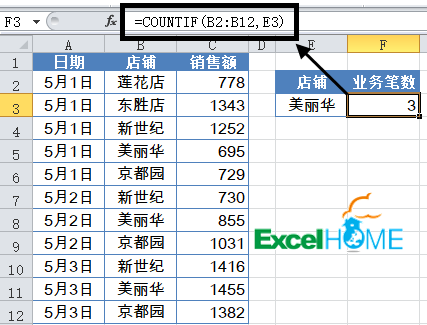COUNTIF函数常规用法为： =COUNTIF(条件区域,指定条件) 统计条件区域中，符合指定条件的单元格个数。 4、根据姓名查询部门 VLOOKUP函数一直是大众情人般的存在，函数的语法为： VLOOKUP(要找谁,在哪儿找,返回第几列的内容,精确找还是近似找) 如下图，要查询F5单元格中的员工姓名是什么职务。 =VLOOKUP($F$5,$B$1:$D$10,2,0) 使用该函数时，需要注意以下几点： 1、第4参数一般用0(或FASLE)以精确匹配方式进行查找。 2、第3参数中的列号，不能理解为工作表中实际的列号，而是指定返回值在查找范围中的第几列。 3、如果查找值与数据区域关键字的数据类型不一致，会返回错误值#N/A。 4、查找值必须位于查询区域中的第一列。 5、根据姓名查询工号 如下图所示，要根据G2单元格姓名，在A~E数据区域中查询对应的工号。 =LOOKUP(1,0/(G2=B2:B6),A2:A6) LOOKUP常用方法为： =LOOKUP(1,0/(条件区域=指定条件),要返回的区域) 6、组合查询 用MATCH函数来定位查询值的位置，再用INDEX函数返回指定区域中指定位置的内容，二者结合，可以实现上下左右全方位的查询。 如下图所示，根据姓名查询部门和职务。 F3单元格公式为： =INDEX(A:A,MATCH($E3,$C:$C,))

7、根据出生年月计算年龄

如下图所示，要根据C列的出生年月计算年龄。

=DATEDIF(C2,TODAY(),"y")

DATEDIF函数第一参数是开始日期，第二参数是结束日期，第三参数是返回的数据类型。

使用Y，表示返回整年数。

使用M，则表示返回整月数。

8、SUMIF多条件求和

要求：统计E2和E3单元格中两个部门的岗位补助总额

公式：

=SUMPRODUCT(SUMIF(B2:B9,E2:E3,C2:C9))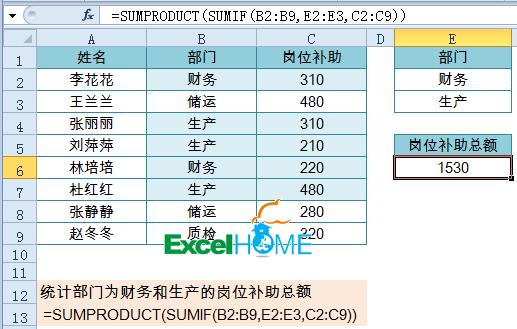SUMIF函数求和条件使用E2:E3，分别得到两个部门的岗位补助额，再使用SUMPRODUCT函数进行求和。

9、SUMIFS多条件求和

要求：统计部门为生产,并且岗位为主操的补助总额

公式：

=SUMIFS(D2:D9,B2:B9,F2,C2:C9,G2)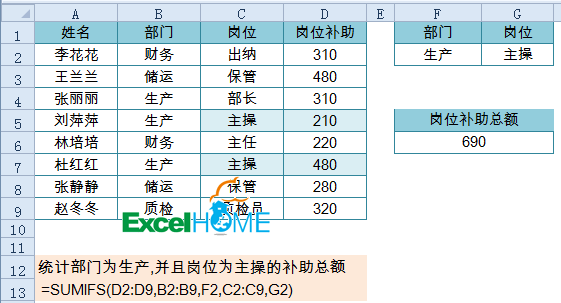SUMIFS函数求和区域为D2:D9，求和条件为B2:B9=F2并且C2:C9=G2

10、包含关键字的多条件求和

要求：统计部门包含“生产”,并且岗位为主操的补助总额

公式：

=SUMIFS(D2:D9,B2:B9,"*"&F2&"*",C2:C9,G2)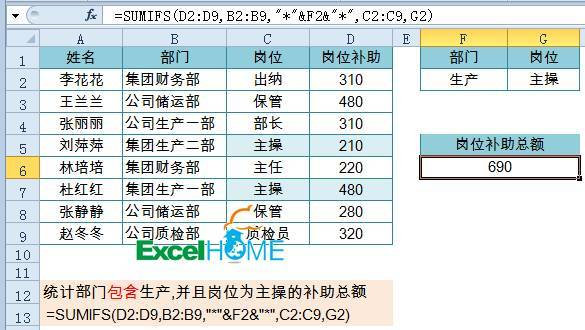SUMIFS函数支持使用通配符。

11、多条件计数

要求：统计统计部门为生产,并且岗位为主操的人数

公式：

=COUNTIFS(B2:B9,F2,C2:C9,G2)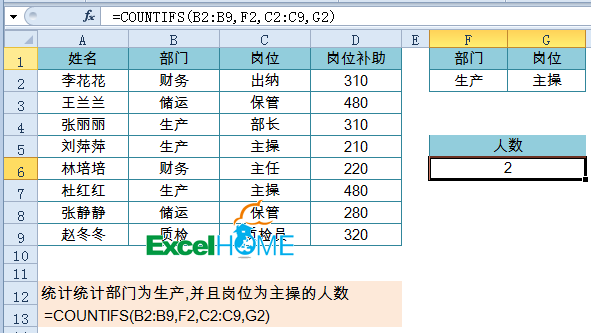COUNTIFS函数也支持使用通配符，用法与SUMIFS函数相同。

12、多条件计算平均值

要求：统计统计部门为“生产”,并且岗位为“主操”的平均补助额

公式：

=AVERAGEIFS(D2:D9,B2:B9,F2,C2:C9,G2)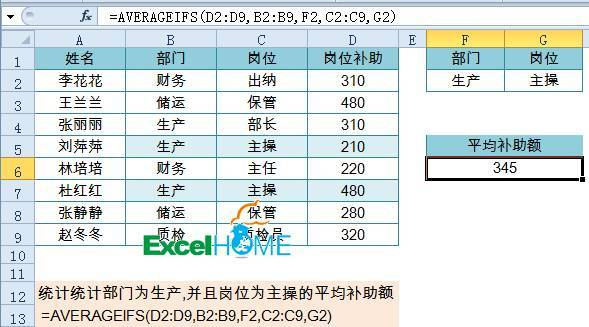第一参数是要统计的数值区域，之后分别是成对的条件区域和指定条件。

13、多条件计算最大值和最小值

要求：统计统计部门为生产,并且岗位为主操的最高补助额

数组公式，注意按Shift+ctrl+回车：

=MAX(IF((B2:B9=F2)*(C2:C9=G2),D2:D9))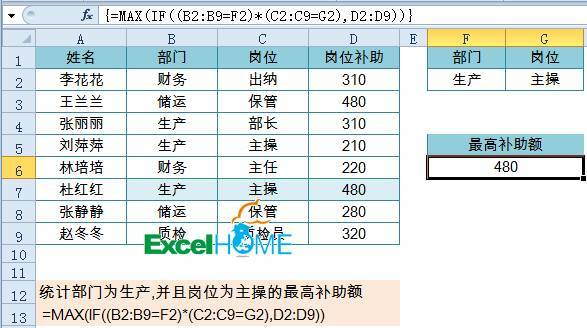数组公式中，判断多条件时不能使用AND或是OR函数，因此先使用两个判断条件相乘，表示两个条件要求同时符合。

再使用IF函数对结果进行判断，两个条件同时符合时，IF函数返回D2:D9中的数值，否则返回逻辑值FALSE。

最后使用MAX函数忽略其中的逻辑值计算出最大值。

要计算多个条件的最小值时，只要将公式中的MAX换成MIN函数即可。

14、根据姓名查询部门

VLOOKUP函数一直是大众情人般的存在，函数的语法为：

VLOOKUP(要找谁,在哪儿找,返回第几列的内容,精确找还是近似找)

如下图，要查询F5单元格中的员工姓名是什么职务。

=VLOOKUP($F$5,$B$1:$D$10,2,0)

使用该函数时，需要注意以下几点：

1、第4参数一般用0(或FASLE)以精确匹配方式进行查找。

2、第3参数中的列号，不能理解为工作表中实际的列号，而是指定返回值在查找范围中的第几列。

3、如果查找值与数据区域关键字的数据类型不一致，会返回错误值#N/A。

4、查找值必须位于查询区域中的第一列。

15、多条件查找

要求：查询部门为生产,并且岗位为部长的姓名

公式：

=LOOKUP(1,0/((B2:B9=F2)*(C2:C9=G2)),A2:A9)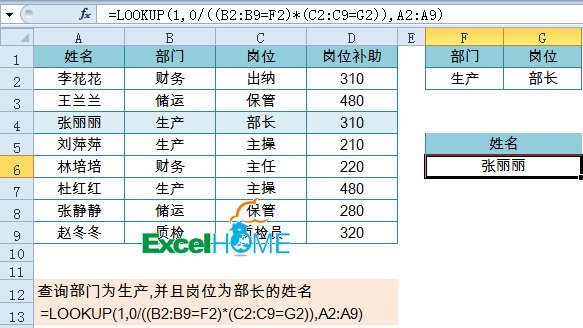LOOKUP函数多条件查询套路为：

=LOOKUP(1,0/(条件1*条件2*条件n),查询区域)

好了，今天的分享就是这些吧，祝各位一天好心情！

责任编辑：

展开全文• 方法：在目标单元格中输入公式：=VLOOKUP(H3,B3:D9,3,0)。 解读： ① 返回B3：D9范围中和H3相匹配的第3列的值。 ② 匹配模式有2种，1为模糊匹配，0位精准匹配。 二、Sumif函数。 功能：单条件求和。 语法：=...

## 使用工具：Microsoft office excel 2016

目录

一、Vlookup函数。

二、Sumif函数。

三、Countif函数。

四、If函数。

五、Sumifs函数。

六、Countifs函数。

七、Round和Int函数。

八、Left、Mid、Right函数。

九、Datedif函数。

十、Iferror函数。

十一、Average和Averageif函数。

十二、Year、Month、Day函数。

十三、Date函数。

十四、Index函数。

十五、Len、Lenb函数。

十六、Match函数。

十七、Mod函数。

十八、Today和Now函数。

十九、Rank函数。

二十、Text函数。

### 一、Vlookup函数。

功能：查找引用。

语法：=Vlookup(查找值，查找范围，返回查找范围中的第几列，匹配模式)。

方法：在目标单元格中输入公式：=VLOOKUP(H3,B3:D9,3,0)。

解读：

① 返回B3：D9范围中和H3相匹配的第3列的值。

② 匹配模式有2种，1为模糊匹配，0位精准匹配。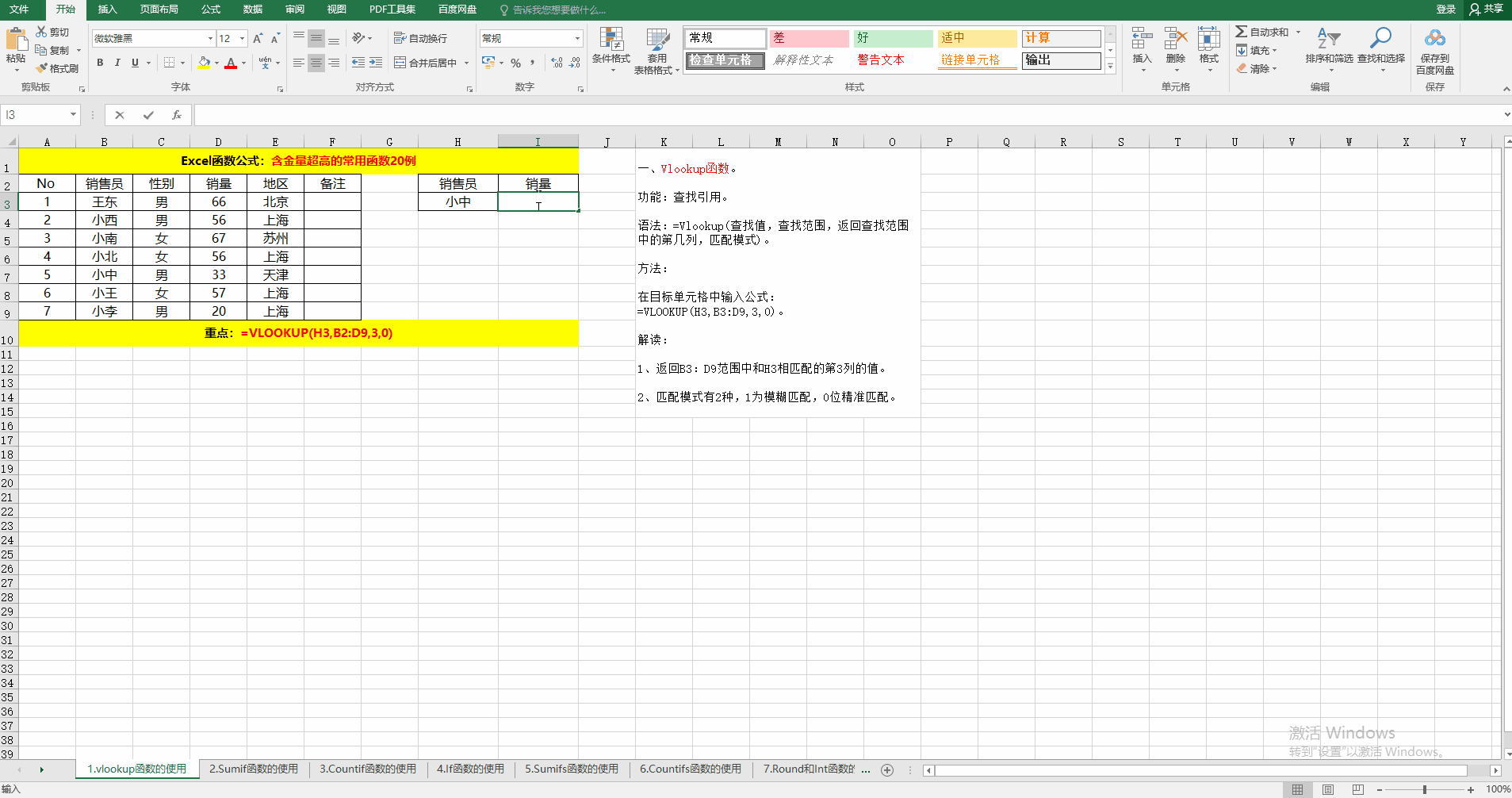### 二、Sumif函数。

功能：单条件求和。

语法：=Sumif（条件范围，条件，求和范围）。

方法：在目标单元格中输入公式：=SUMIF(C3:C9,H3,D3:D9)。

解读：求和的目的在于：统计男or女销售员的销量和。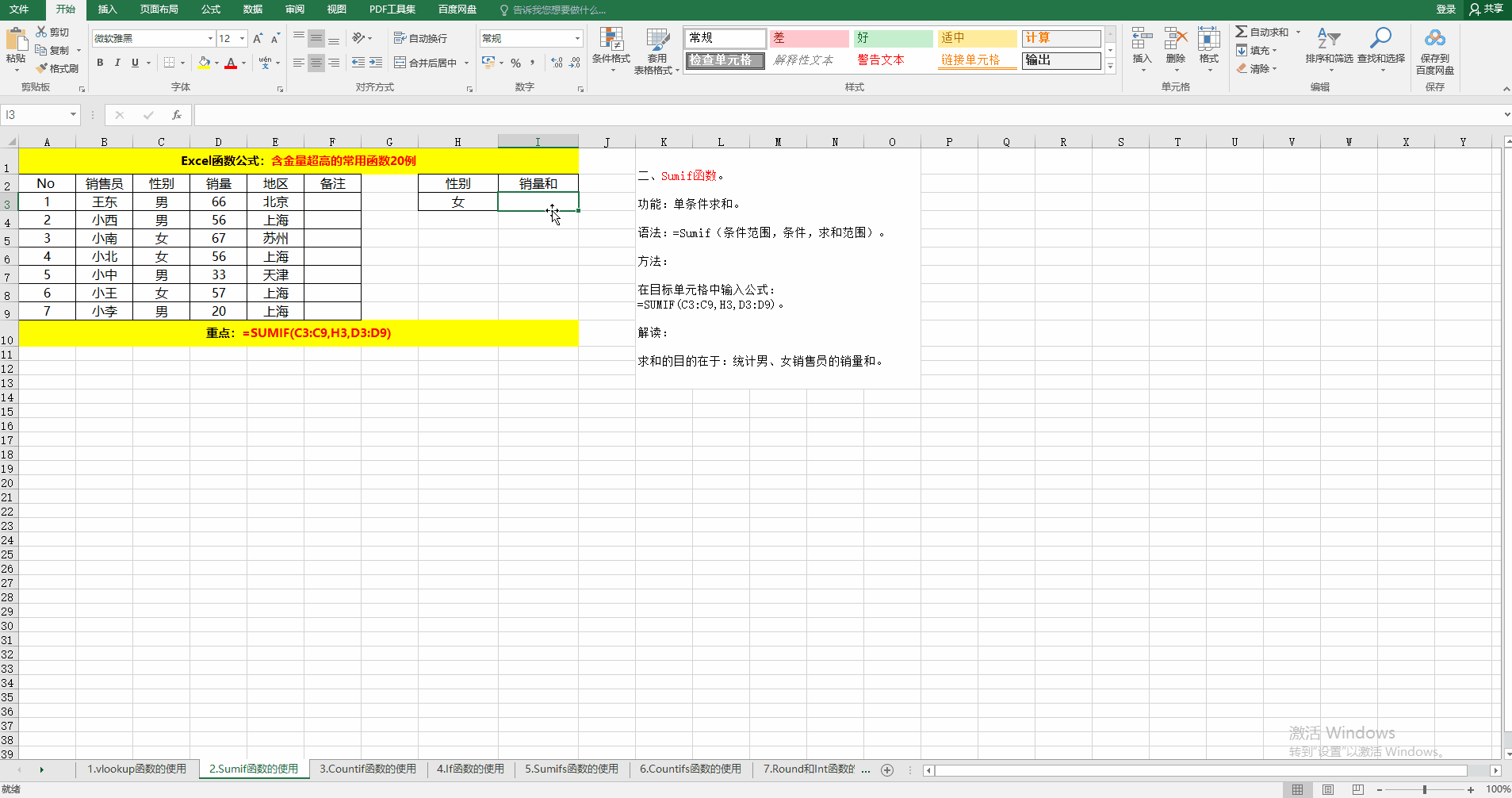### 三、Countif函数。

功能：条件计数。

语法：=Countif(条件范围，条件)。

方法：在目标单元格中输入公式：=COUNTIF(C3:C9,H3)。

解读：示例的目的在于统计男or女销售员的人数。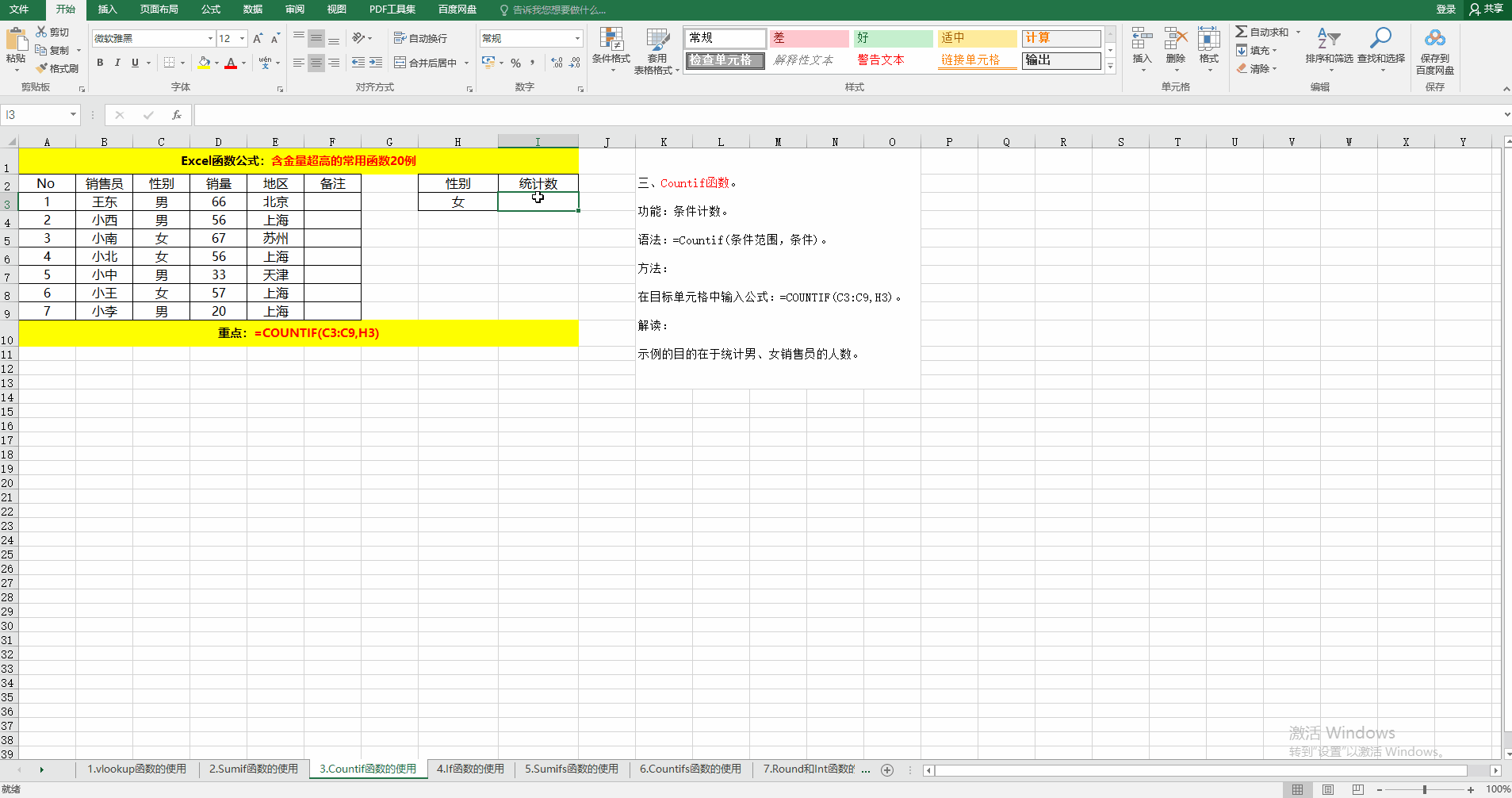### 四、If函数。

功能：根据条件判断结果返回相应的值或公式的计算结果。

语法：=If(判断条件，条件为真时返回的值，条件为假时返回的值）。

方法：在目标单元格中输入公式：=IF(D3>=60,"是","否")。

解读：如果销量大于等于60，则返回"是"，否则返回"否"。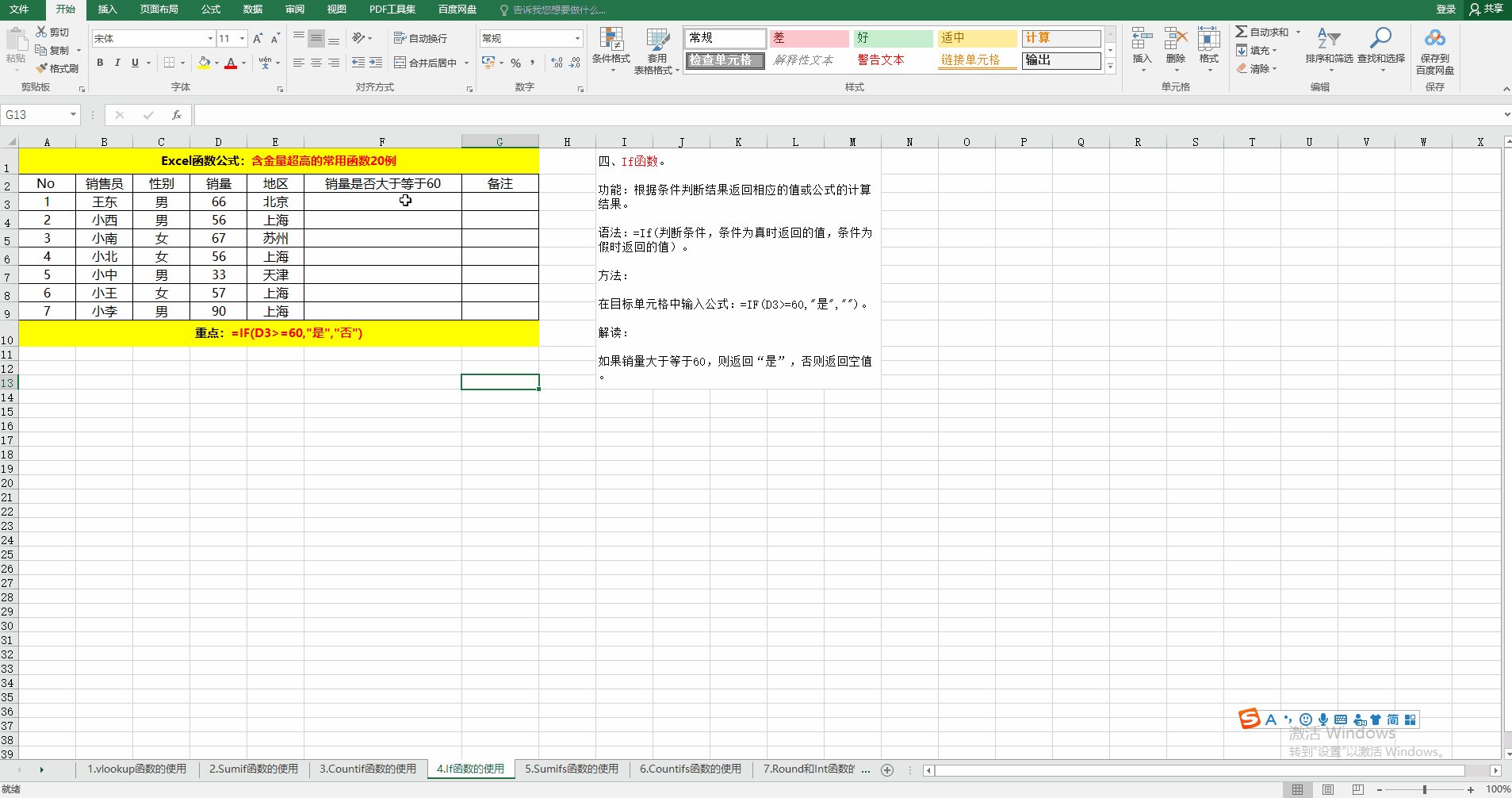### 五、Sumifs函数。

功能：多条件求和。

语法：=Sumifs(求和范围，条件1范围，条件1,……条件N范围，条件N)。

方法：在目标单元格中输入公式：=SUMIFS(D$3:D$9,C$3:C$9,H3,D$3:D$9,I3)。

解读：求男、女销售员销量大于等于60的销量和。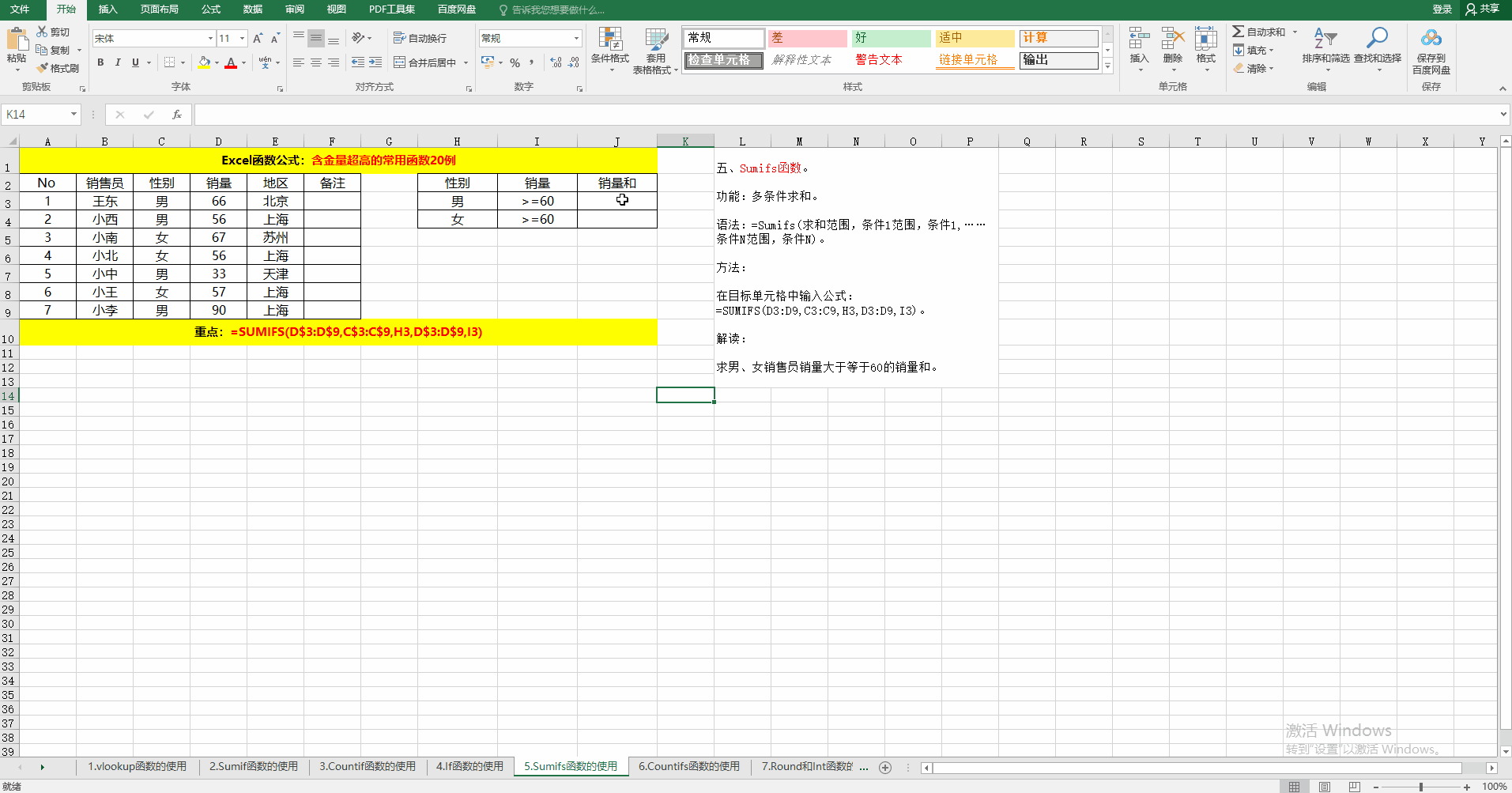### 六、Countifs函数。

功能：多条件计数。

语法：=Countifs(条件1范围，条件1……条件N范围，条件N）。

方法：在目标单元格中输入公式：=COUNTIFS(C$3:C$9,H3,D$3:D$9,I3)。

解读：求男、女销售员销量大于等于60的人数。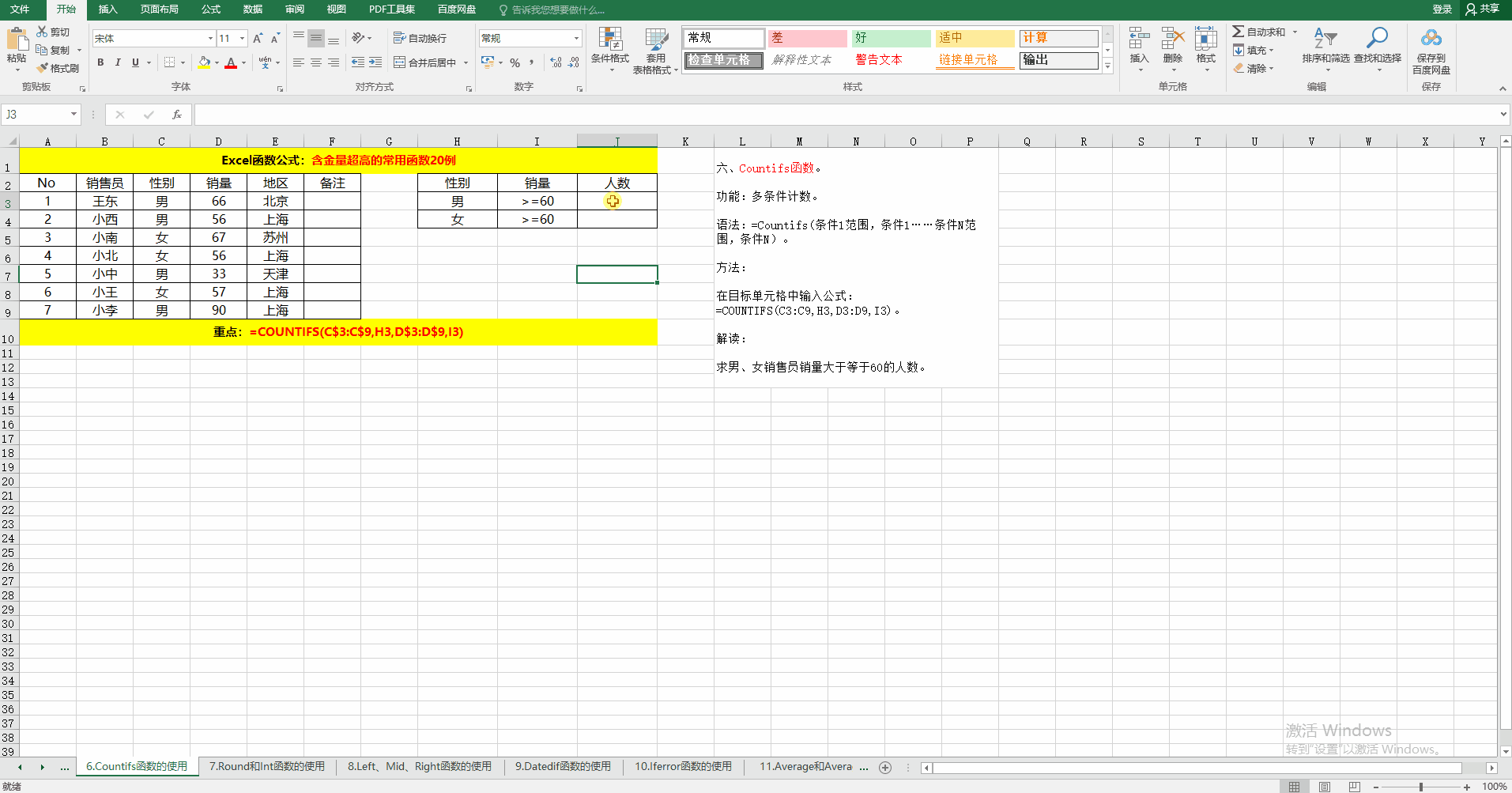### 七、Round和Int函数。

功能：四舍五入、取整。

语法：=Round（值，保留位数）；=Int（值）。

方法：在目标单元格中输入公式：=ROUND(I3,1)、=INT(I3)。

解读：Round函数根据保留位数四舍五入。而Int函数直接取整数位，不进行四舍五入。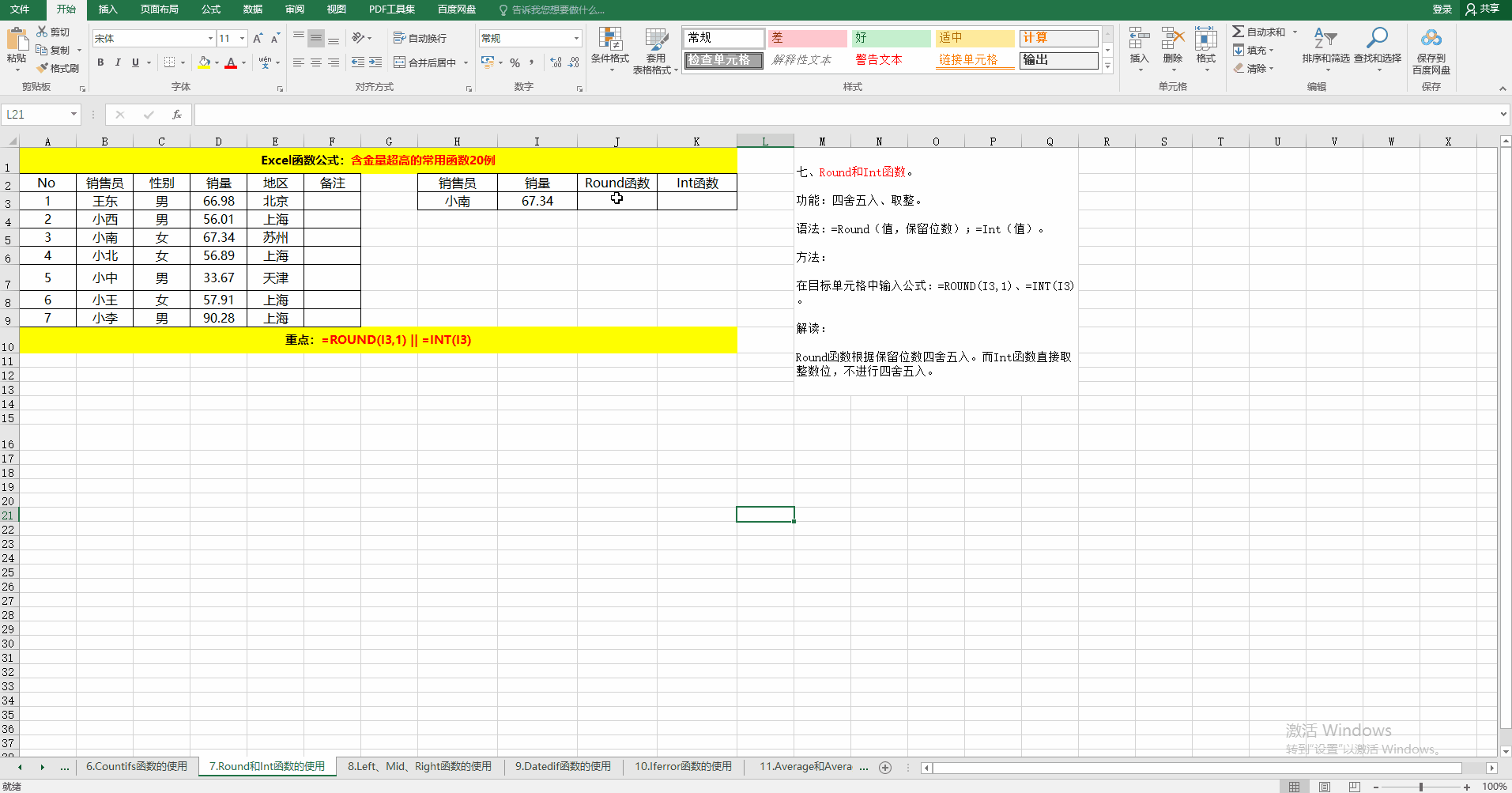### 八、Left、Mid、Right函数。

功能：提取指定的字符串。

语法：

① =Left(字符串，从左边开始提取的字符串位数)。

② =Mid(字符串，开始字符串的位置，需要截取的长度)。

③ =Right(字符串，从右边开始提取的字符串位数)。

方法：在目标单元格中输入公式：=LEFT(F3,4)、=MID(F3,5,5)、=RIGHT(F3,4)。

解读：从指定的字符串中提取指定的字符串。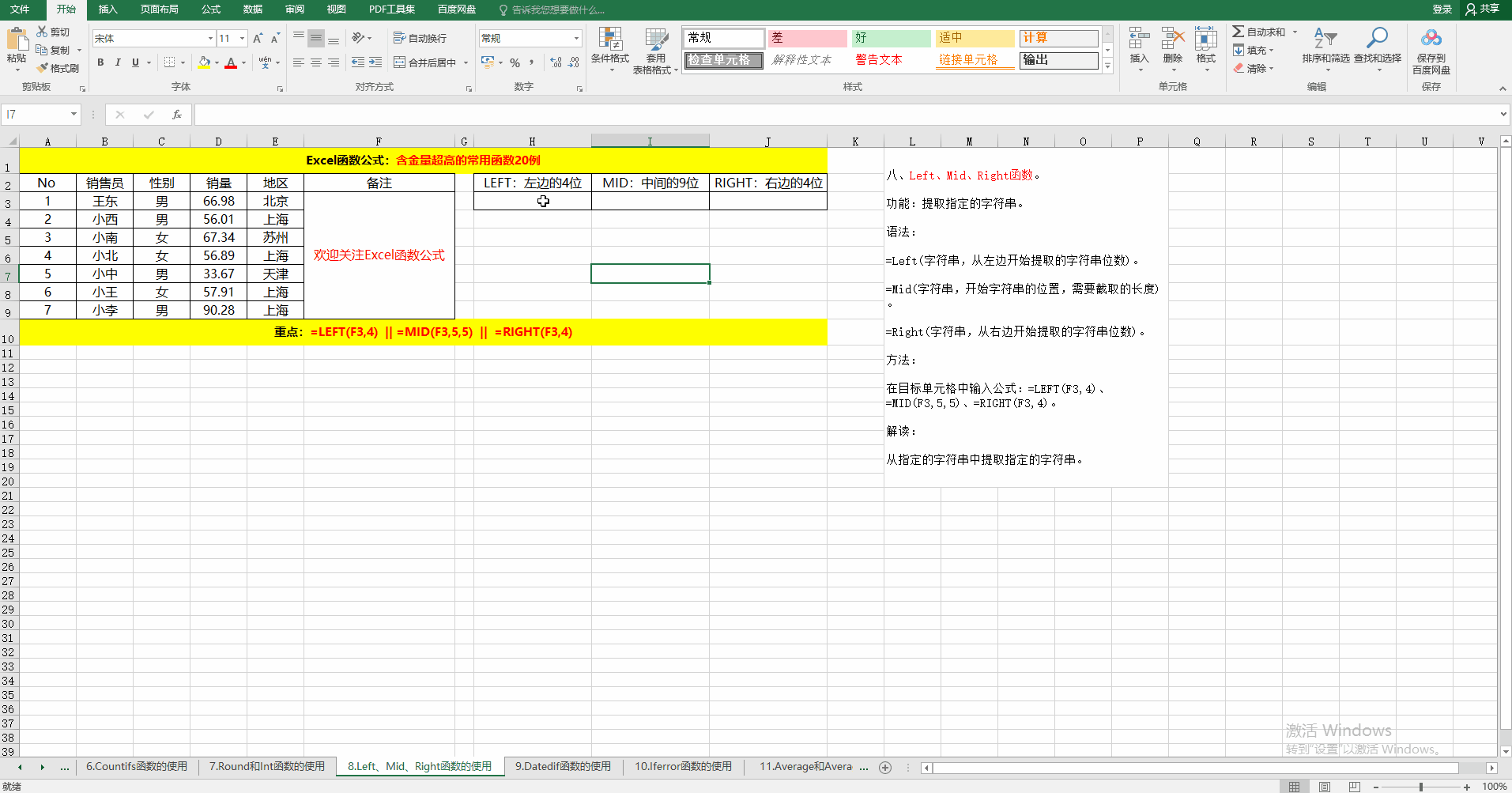### 九、Datedif函数。

功能：按指定的统计方式统计时间差。

语法：=Datedif(开始时间，结束时间，统计方式)。

方法：在对应的目标单元格中输入公式：=DATEDIF(C3,D3,"y")、=DATEDIF(C3,D3,"m")、=DATEDIF(C3,D3,"d")。

解读：Datedif函数为系统隐藏函数，其统计方式有：Y（年）、M（月）、D（日）、MD（忽略年和日的天数）、YM（忽略年和日的月数）、YD（忽略年的日的天数）。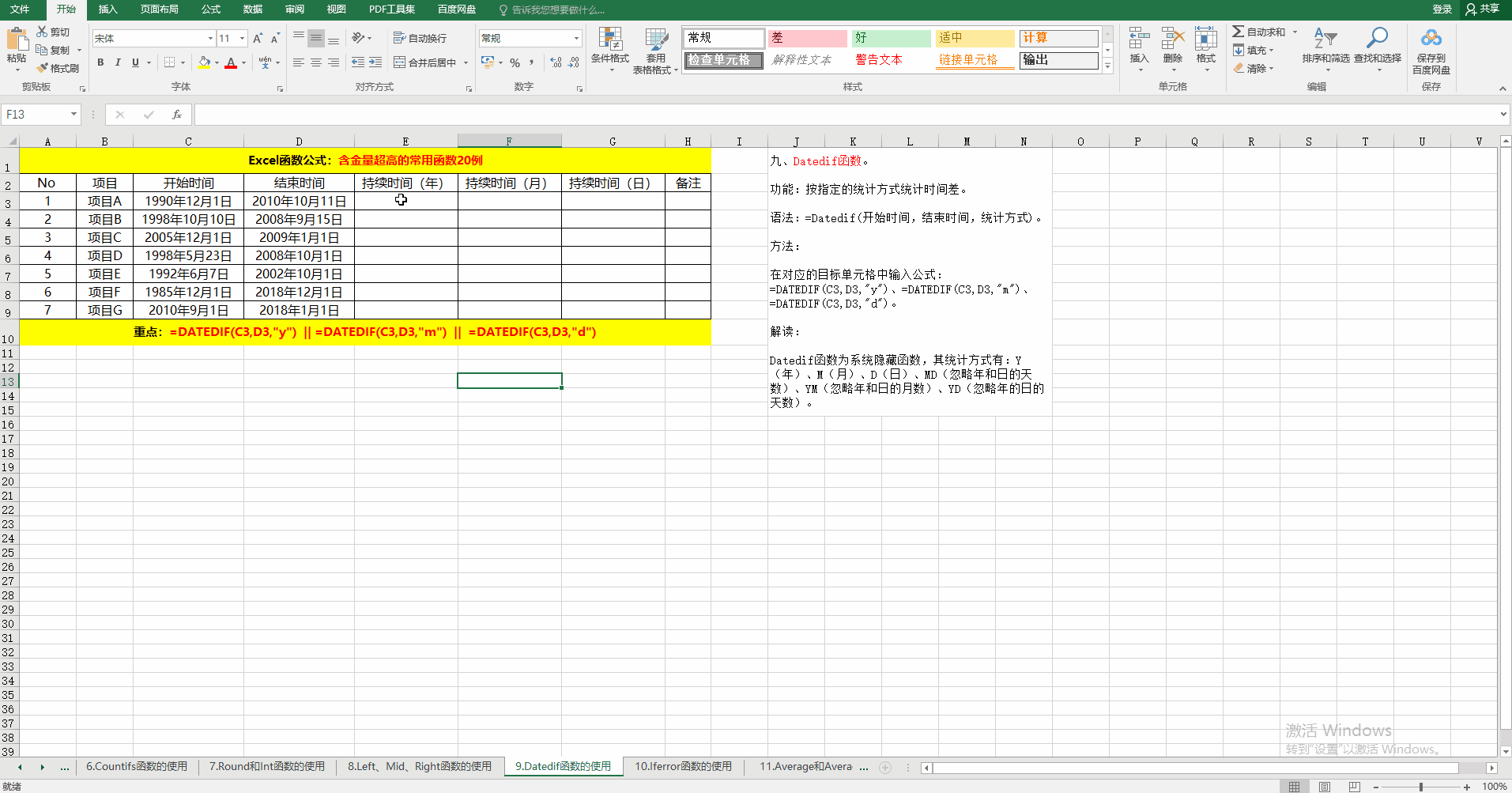### 十、Iferror函数。

功能：判断表达式或公式是否有错误，如果有错误，则返回指定的值，否则返回表达式或公式本身的值。

语法：=Iferror(表达式或公式，公式错误时返回的值)。

解读：除数不能为0，当除数为0时返回错误。利用Iferror返回空值。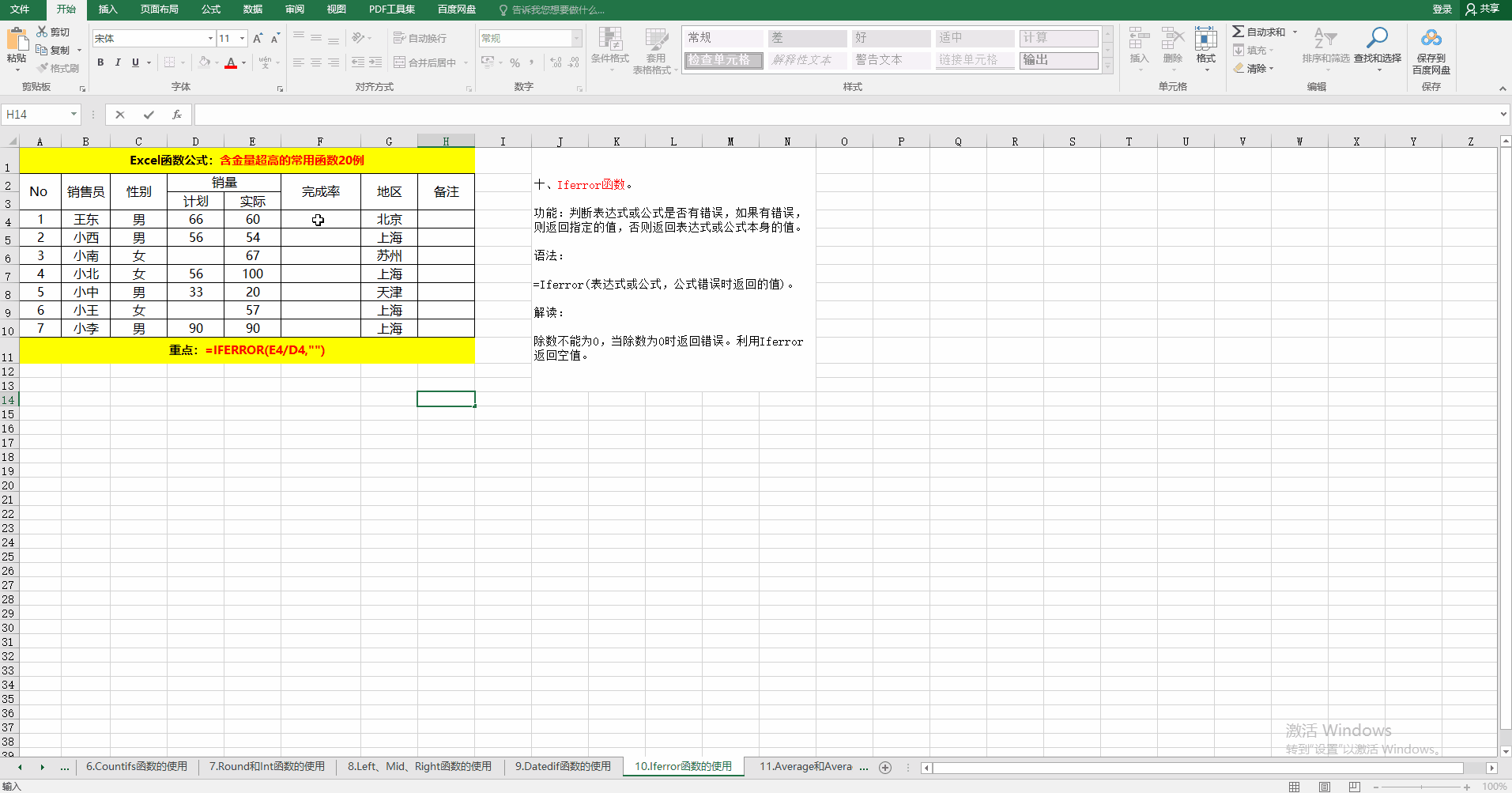### 十一、Average和Averageif函数。

功能：计算平均值或根据指定的条件计算平均值。

语法：

① =Average(值范围)。

② =Averageif(条件范围，条件，值范围）。当条件范围和值范围相同时，值范围可以省略哦！

方法：在目标单元格中输入公式：=AVERAGE(D3:D9)、=AVERAGEIF(C3:C9,H4,D3:D9)。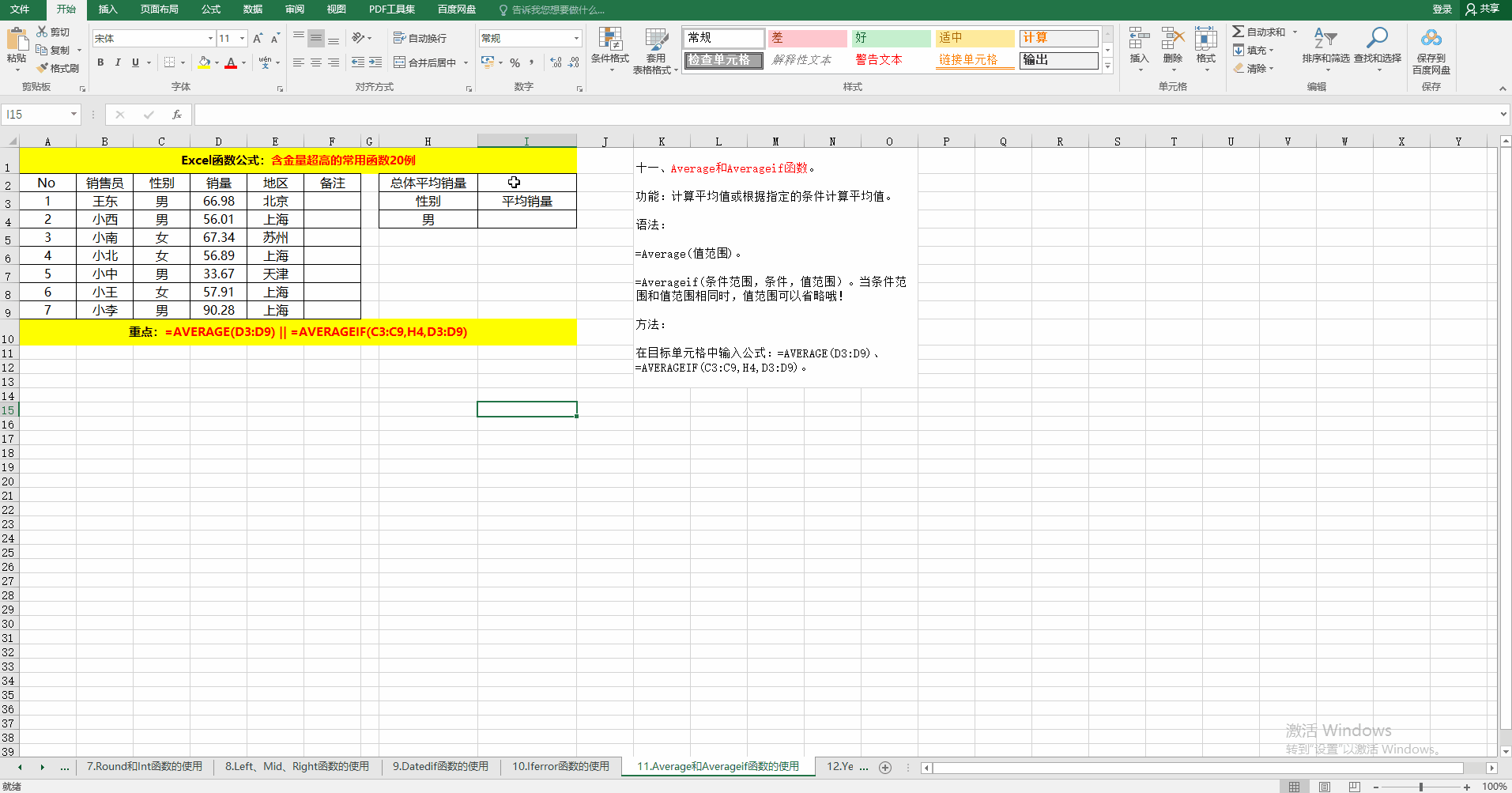### 十二、Year、Month、Day函数。

作用：提取日期中的年（Year）、月（Month）、日（Day）。

语法：=Year（日期）；=Month（日期）；=Day（日期）。

方法：在目标单元格中输入公式：=YEAR(C3)、=MONTH(C3)、=DAY(C3)。

解读：利用函数Year、Month、Day可以提取日期中对应的值，也可以利用Date将数值组成日期。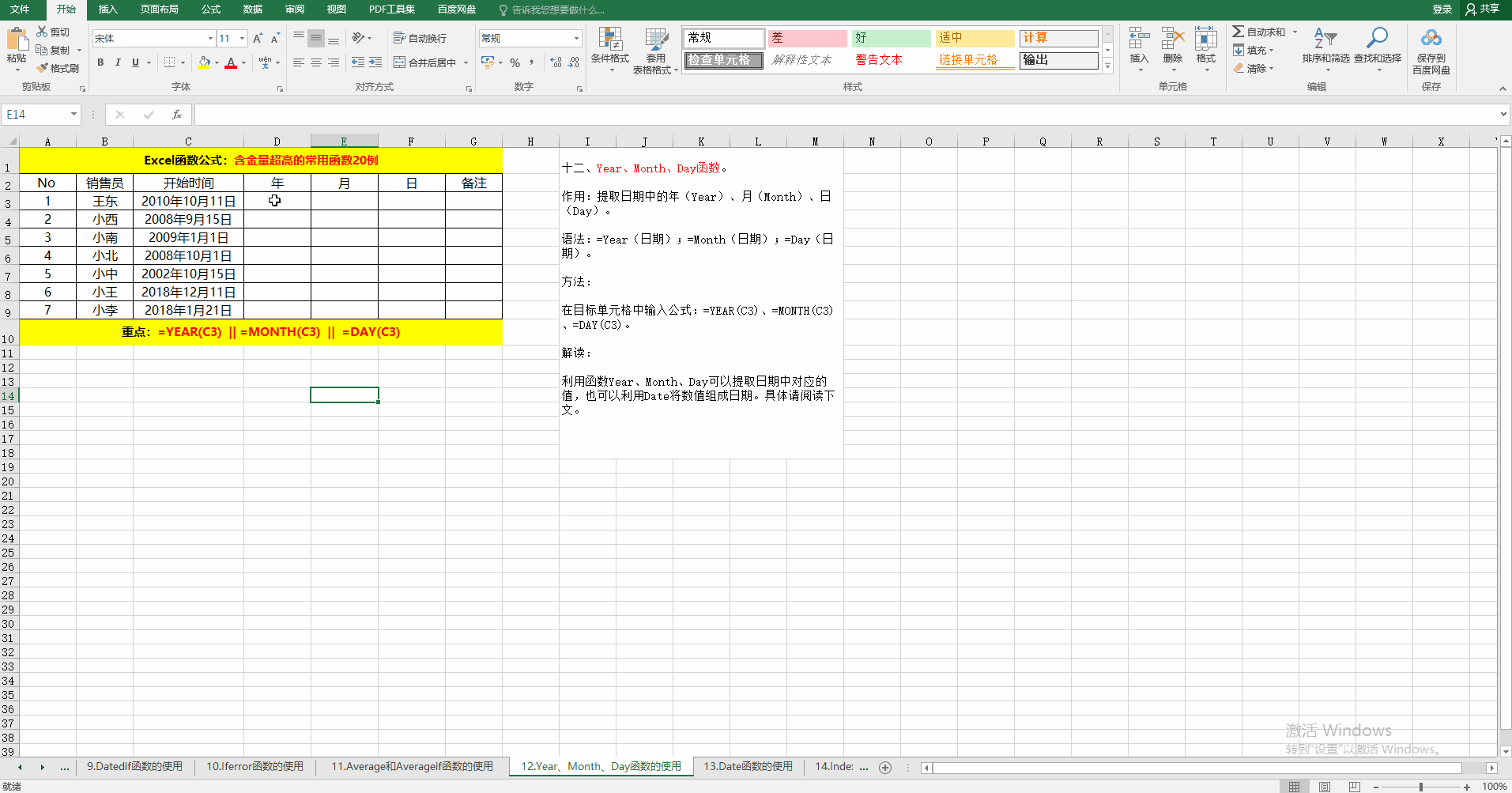### 十三、Date函数。

作用：将指定的值转换为日期。

语法：=Date（表示年的值，表示月的值，表示日的值）。

方法：在目标单元格中输入公式：=DATE(C3,D3,E3)。

解读：

①未转换之前单元格的格式为“常规”。

②转换后单元格的格式变为“日期”，自动变化哦！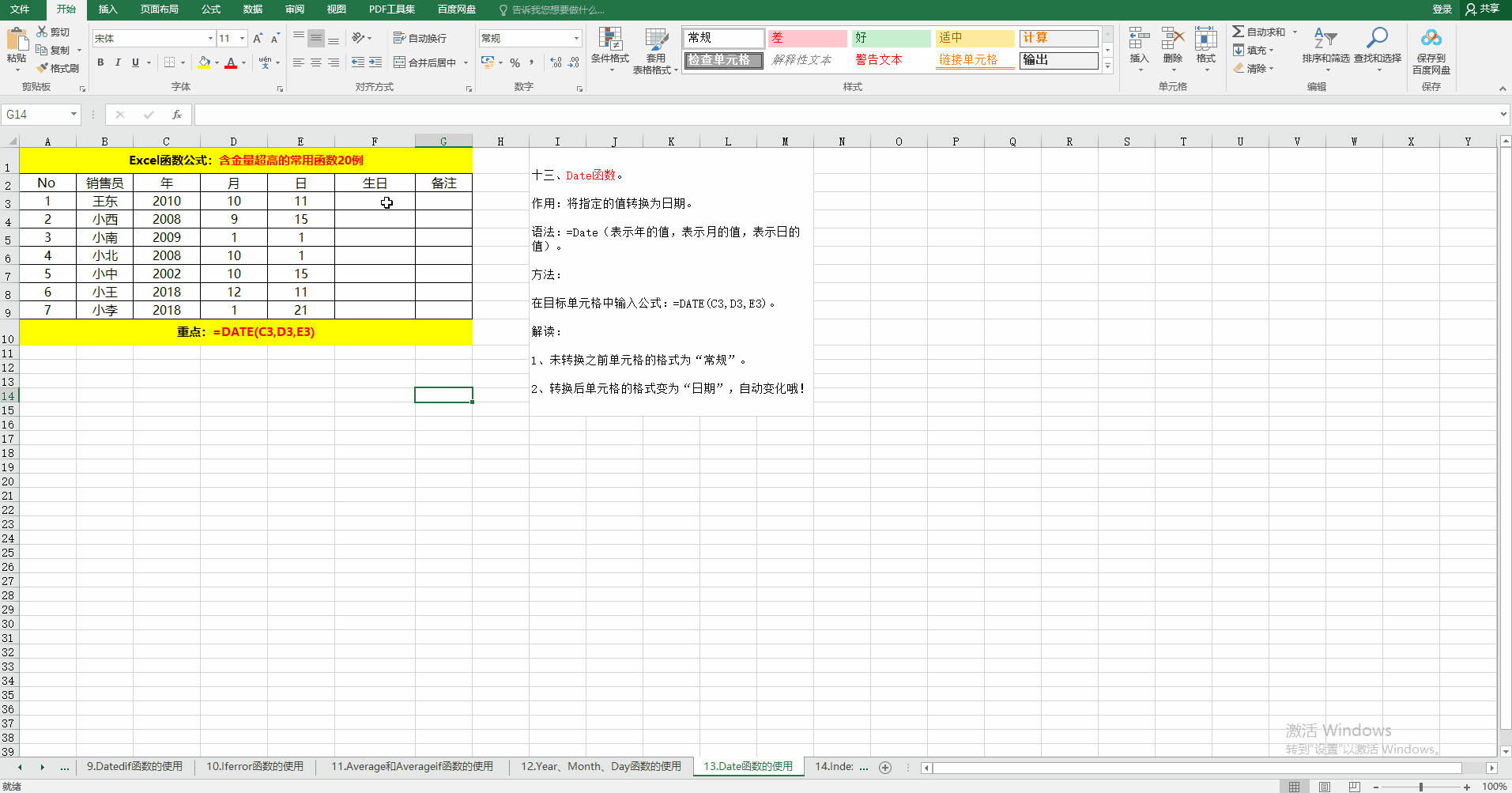### 十四、Index函数。

作用：返回列表或数组中指定位置的值。

语法：=Index(数据区域，行，列)。

方法：在目标单元格中输入公式：=INDEX(B3:E9,3,4)。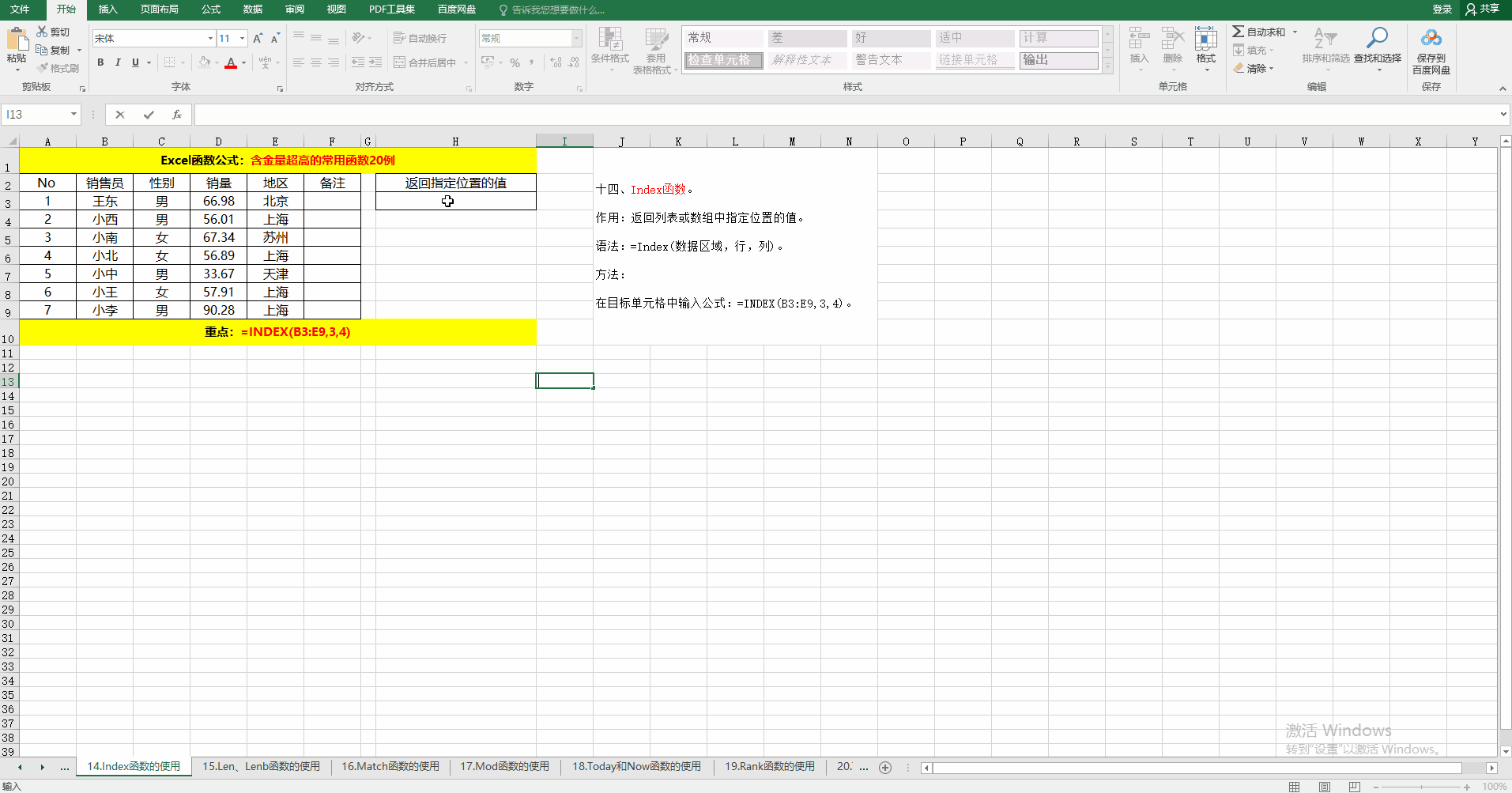### 十五、Len、Lenb函数。

作用：返回字符串中的字符串和字节数。

语法：=Len(字符串)、=Lenb(字符串)。

方法：在目标个输入公式：=LEN(A1)、=LENB(A1)。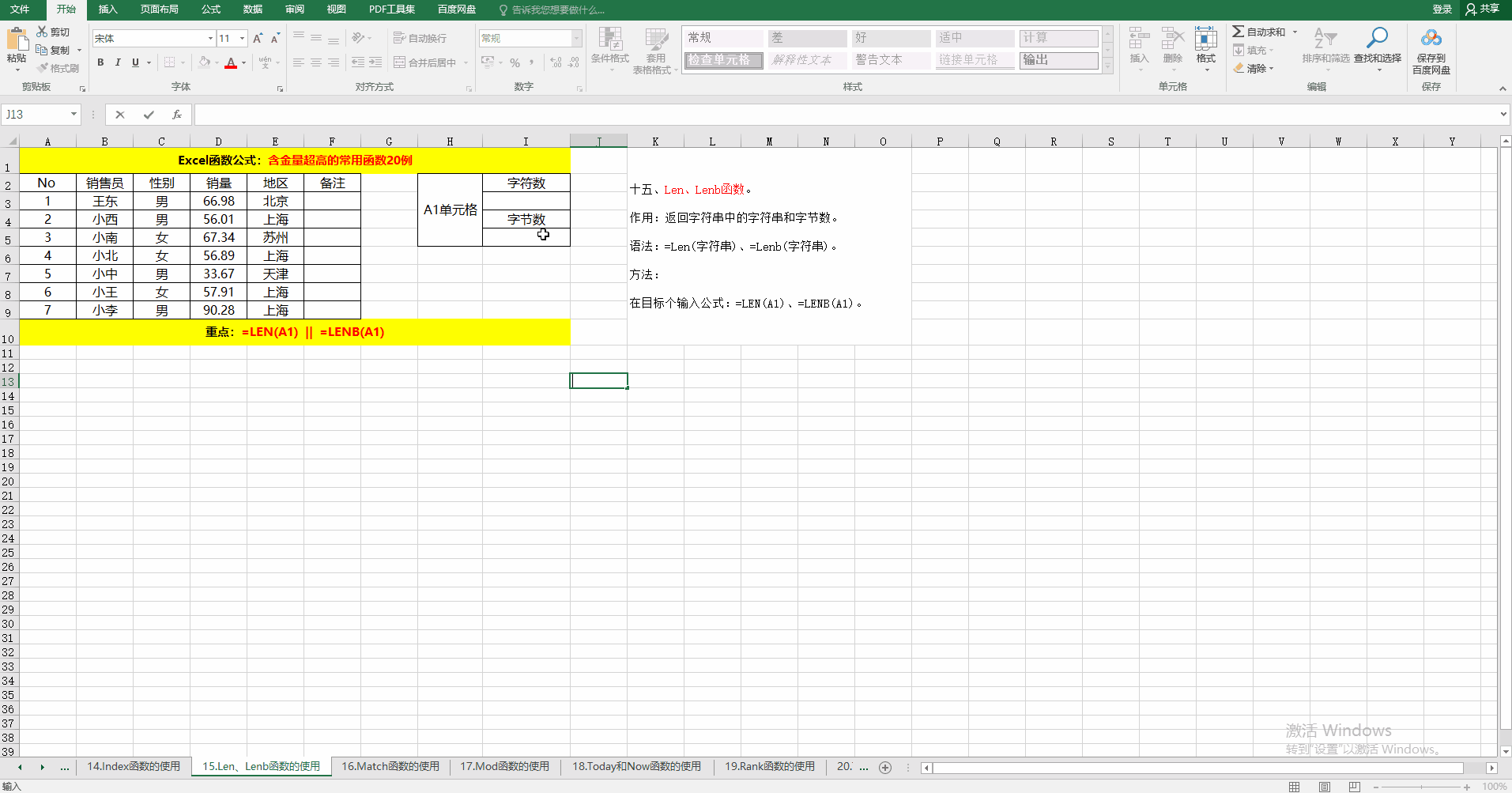### 十六、Match函数。

作用：返回指定方式下与指定值匹配的数组元素中元素的位置。

语法：

=Match(查找值，查找值所在的返回，匹配方式)。

其中匹配方式为：-1,0,1。其所表示的意义分别为：

-1：查找大于或等于查找值的最小值，查找范围必须按降序排列。

0：精准查找，查找范围可以按任何顺序排列，如果省略第三个参数，默认为0.

1：查找小于或等于查找值的最大值，查找范围必须按升序排列。

方法：在目标单元格中输入公式：=MATCH(H3,B3:B9,0)。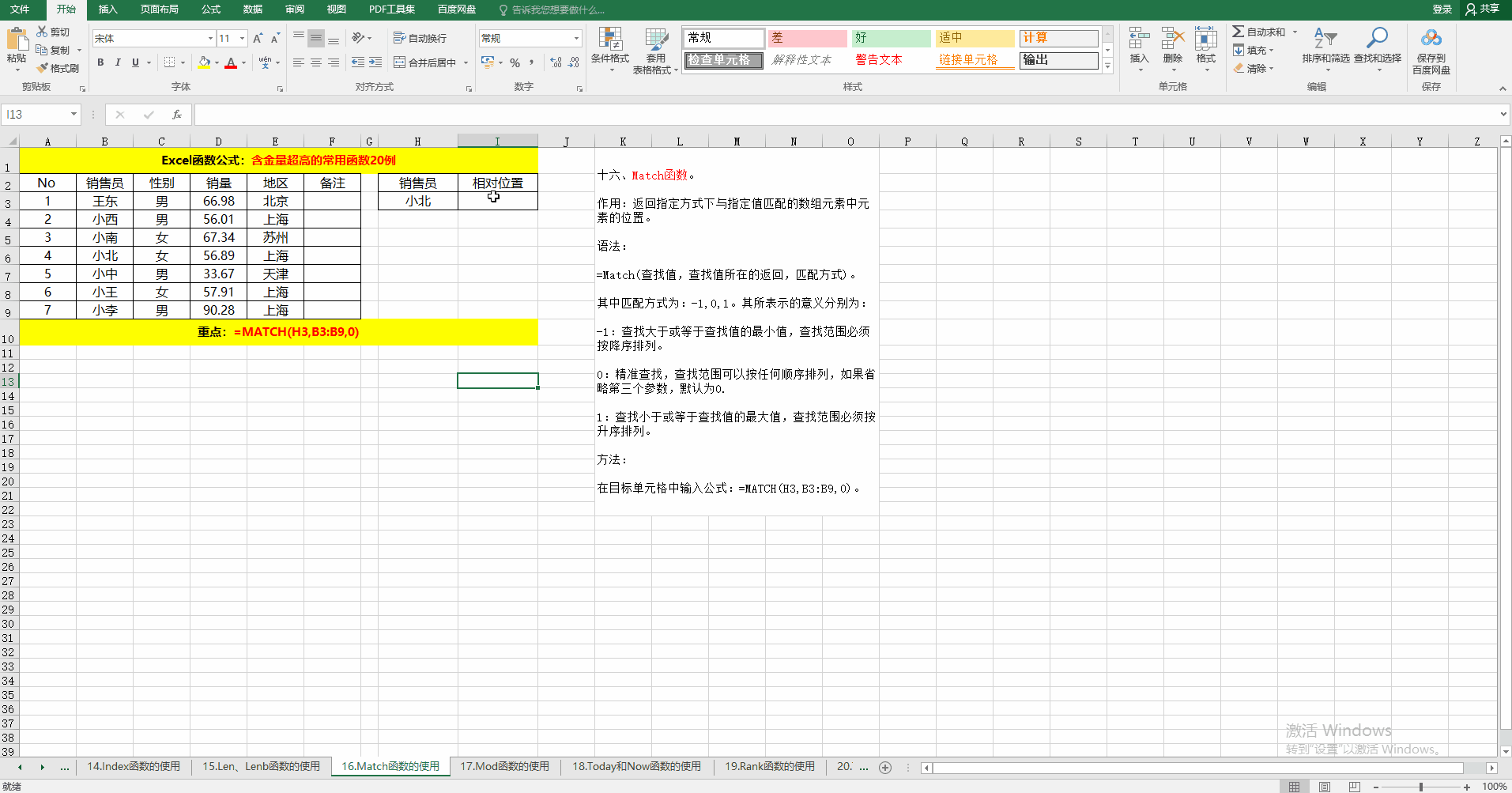### 十七、Mod函数。

作用：计算两个数相除之后的余数。

语法：=Mod(被除数，除数)。

方法：在目标单元格中输入公式：=MOD(B3,C3)。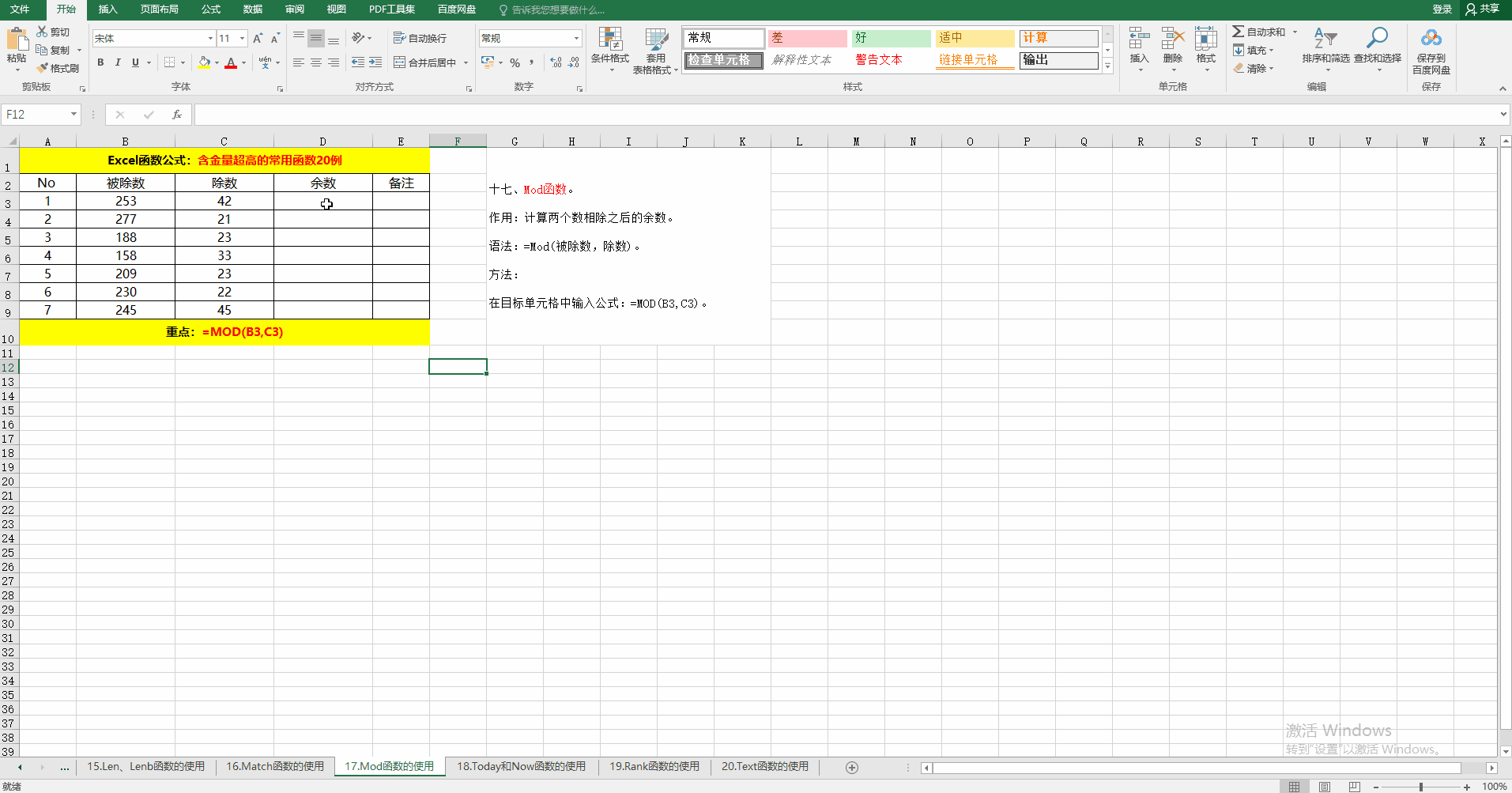### 十八、Today和Now函数。

作用：获取系统当前日期和时间。

语法：=Today() 、=Now()。

方法：在目标单元格中输入公式或按相应的快捷键。

解读：获取系统日期和时间除了用函数之外，还可以实用快捷键，分别为：Ctrl + ; 和 Ctrl+Shift + ; 。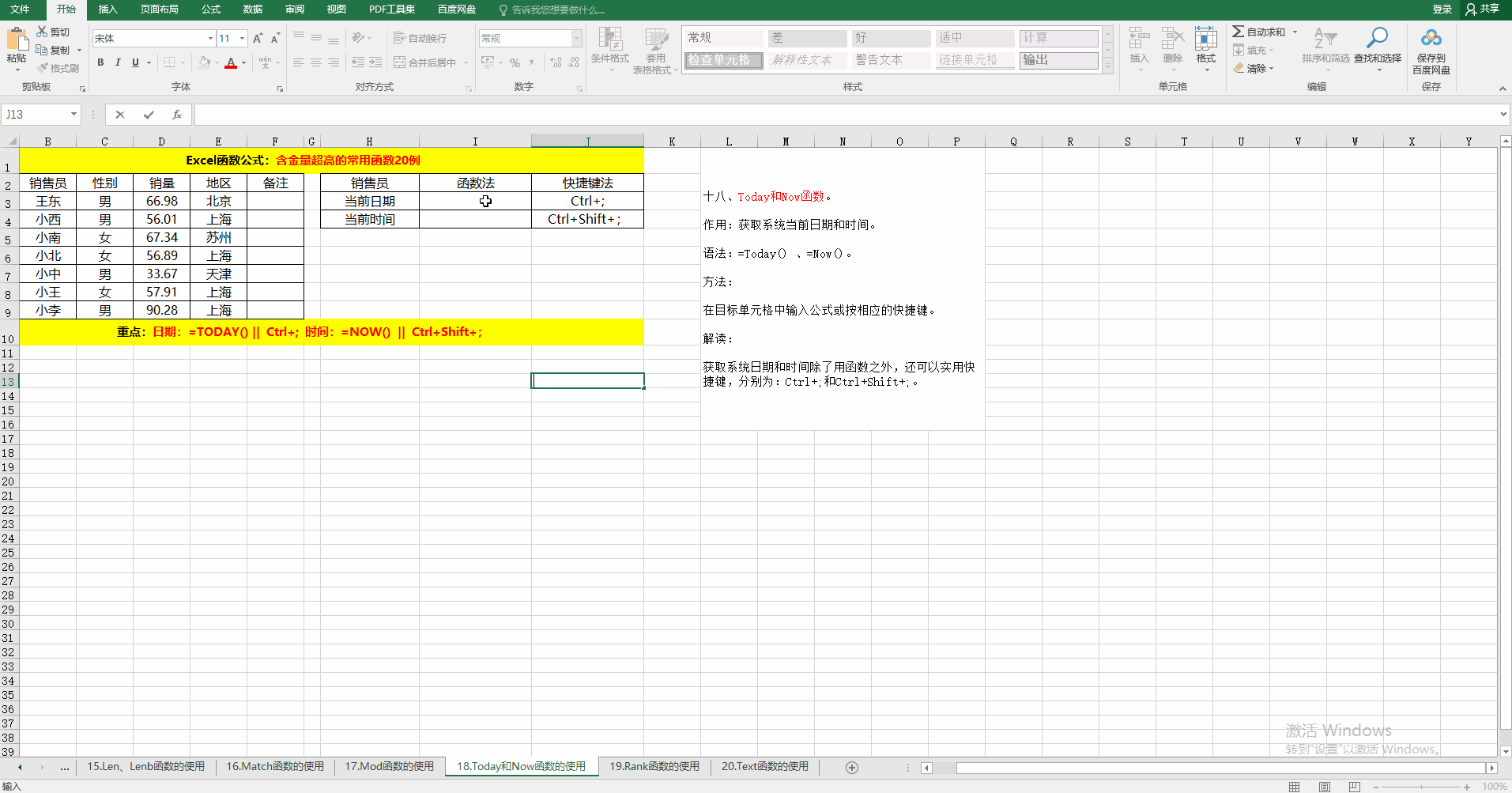### 十九、Rank函数。

作用：对给定的值按照指定的顺序排序。

语法：=Rank(排序的值，排序范围，排序方式)。

排序方式有1和0两种，1为升序，0位默认值，降序。

方法：

在目标单元格中输入公式：

① 升序=RANK(D3,D$3:D$9,1)

② 降序=RANK(D3,D$3:D$9,0)或=RANK(D3,D$3:D$9)。

解读：当省略第三个参数时，默认为降序排序，和第三个参数为0的效果相同哦！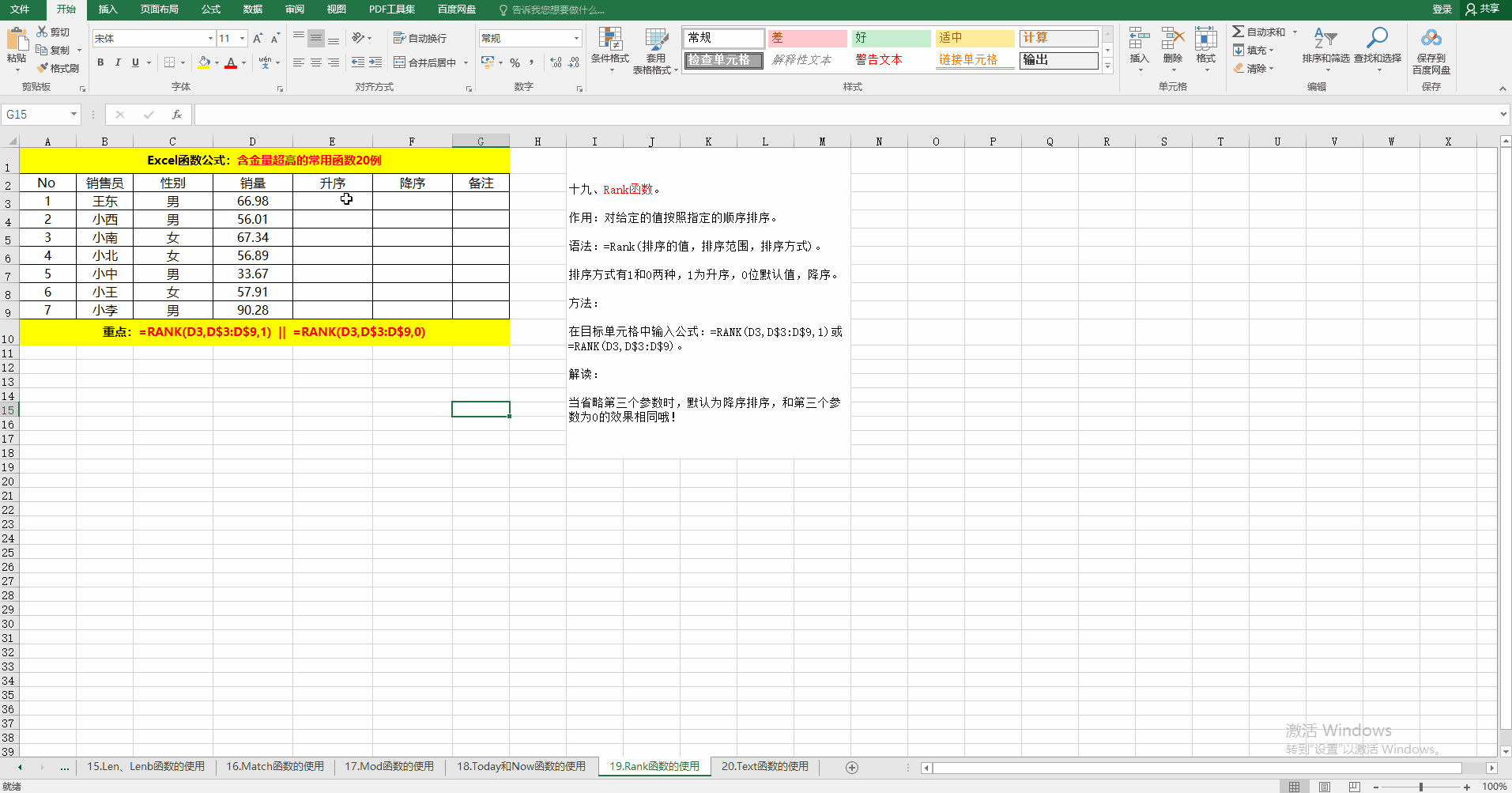### 二十、Text函数。

作用：根据指定的代码将相应的值设置为相应的格式。

语法：=Text(值，格式)。

方法：在目标单元格中输入公式：=TEXT(D3,"$0.00")。 解读：示例中将数字转换为货币形式，当然还可以转换为其它形式哦。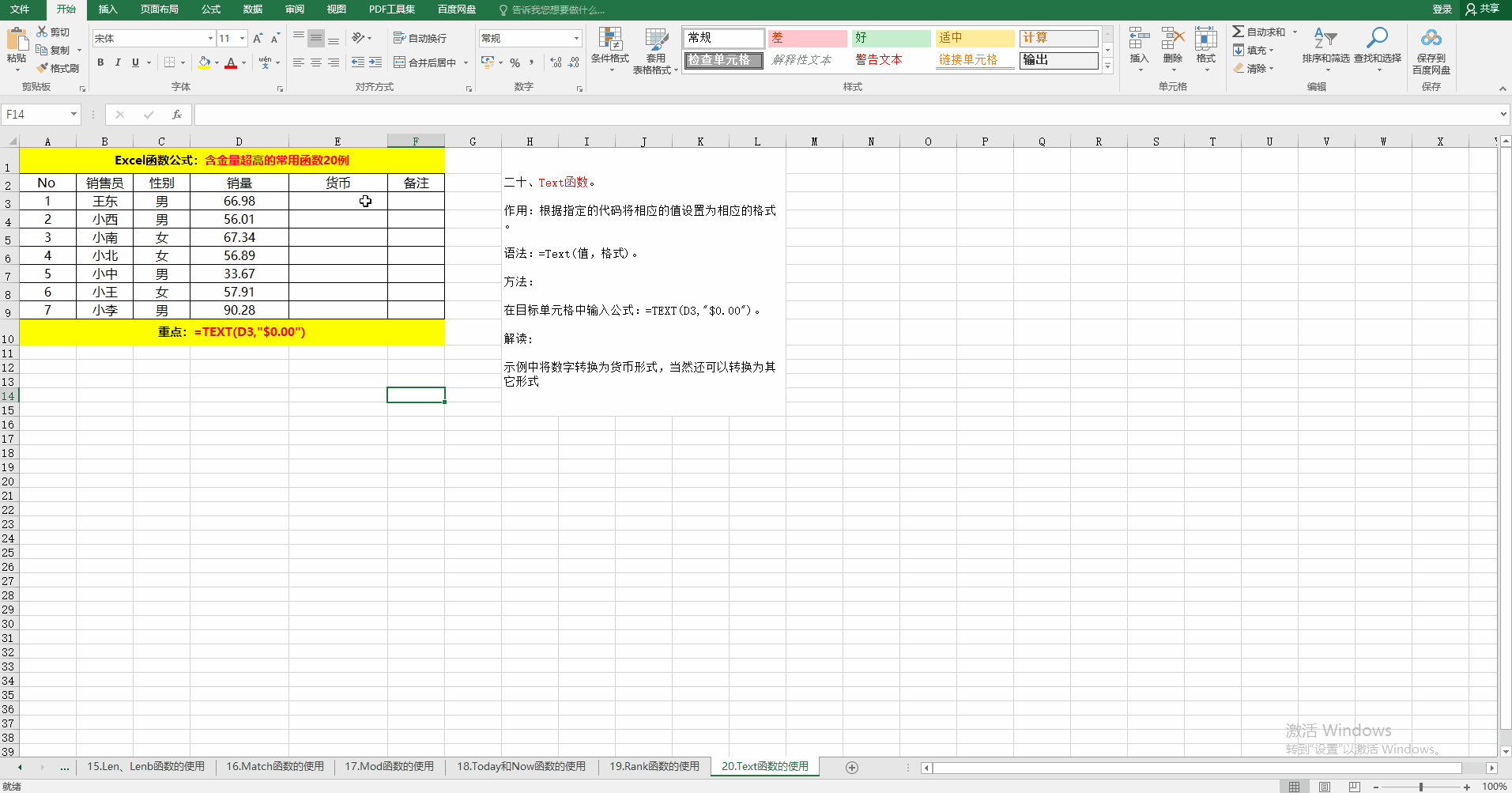附：Excel常用函数公式样例下载地址：https://github.com/13714791953/Excel2016.git 展开全文excel • excel是一款非常好用的表格数据...excel常用函数大全1、SUM函数：SUM函数的作用是求和。函数公式为=sum()例如：统计一个单元格区域：=sum(A1:A10) 统计多个单元格区域：=sum(A1:A10,C1:C10)2、AVERAGE函数:Averag... •文档 •文档 • 计算机二级考试04-06 22:26Excel一共提供了数百个内部函数，但计算机二级常考的和工作中常用的，下图已经帮你们整理好了！除公式外，还配上了详细讲解＋考题示例，让你在实例中掌握要点！ 学生党和职场人士必备，... •文档 • 1、基础汇总公式A列求和：=SUM(A:A)A列最小值：=MIN(A:A)A列最大值：=MAX (A:A)A列平均值：=AVERAGE(A:A)A列数值个数：=COUNT(A:A)2、成绩排名=RANK.EQ(A2,A$2:A\$7)3、中国式排名(相同成绩不占用名次)=SUMPRODUCT((B...
• 计算机二级 Excel常用函数公式总结 -复习笔记求和函数求平均函数最大值/最小值函数求个数函数排名函数逻辑判断函数（多）条件求个数函数（多）条件求和函数乘积求和函数查询函数index函数match函数left/right函数mid...excel
•文档
•文档
•语言
• 一、数学函数 1.取整 =INT(数字) 2.求余数 =MOD(除数，被除数) 3.四舍五入 =ROUND（数字，保留小数位数） 4.取绝对值 =ABS(数字) 5.算术平方根 =SQRT(数字) 6.产生随机数 =RAND() 7.返回一个介于指定的数字...excel
• 批量插入公式批量修改公式快速查找公式显示公式部分计算结果保护公式隐藏公式显示所有公式公式转换成值显示另一个单元格的公式公式表达式转换为结果快速查找公式中的错误1批量输入公式选取要输入的区域，在编辑...
•文档
• 今天和大家分享的这些Excel函数都是最基本的，但应用面却非常广，学会基本Excel函数，也可以让工作事半功倍。1、SUM函数SUM函数的作用是求和。统计一个单元格区域：=sum(A1:A10)统计多个单元格区域：=sum(A1:A10,C1:...
•EXCEL...